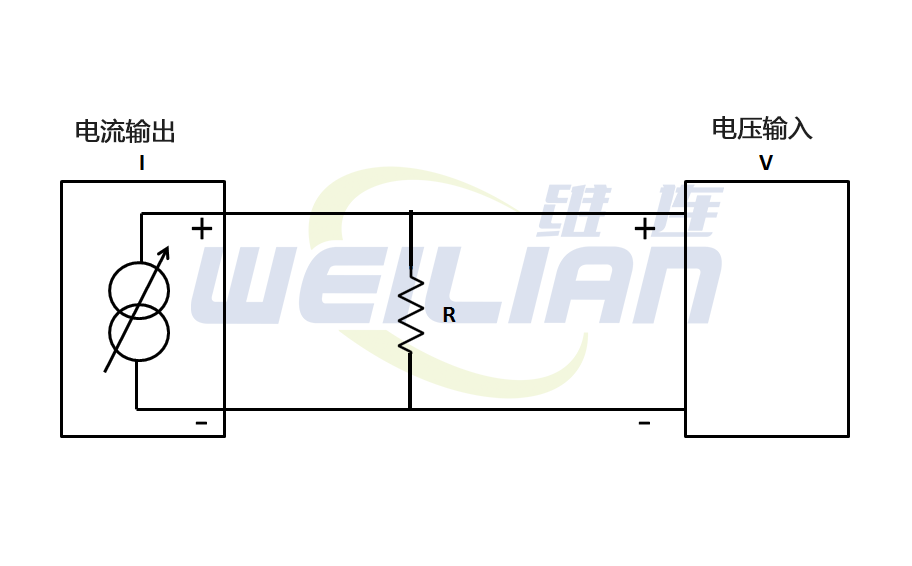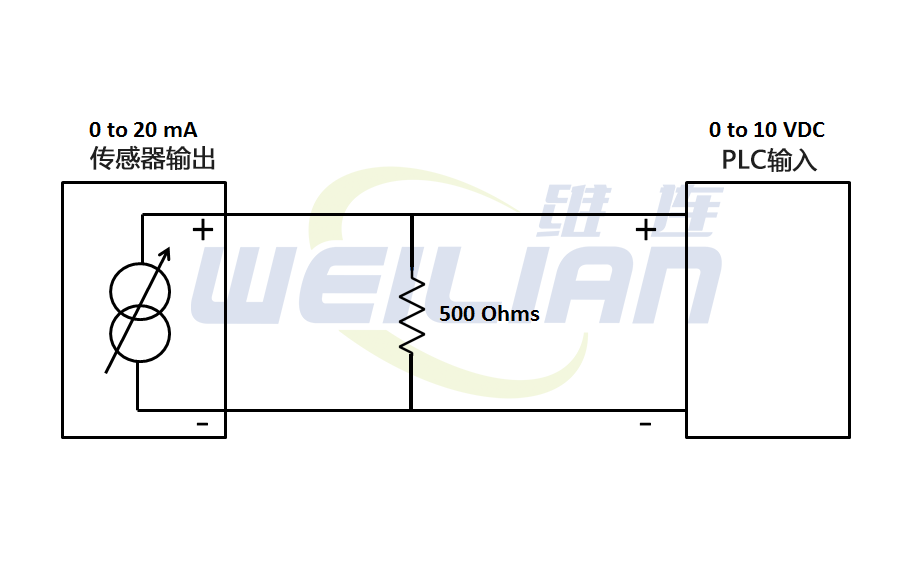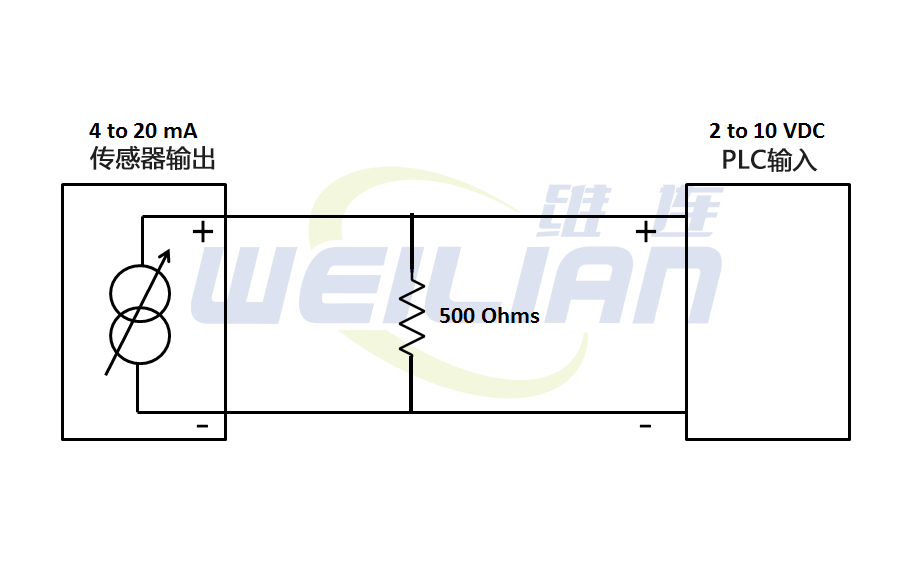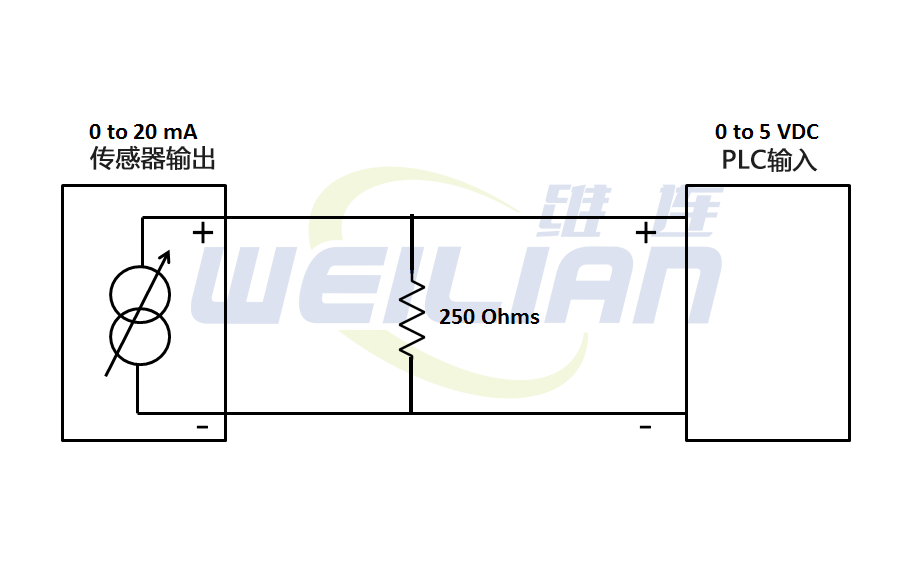### 如何使用电阻将电流转换为电压？

2021-09-14       来源：www.weilianchina.comR = 10V/0.020A = 500 欧姆V = I*R = 0*500 = 0V

V = I*R = 0.020*500 = 10V

R = 10V/0.020A = 500 欧姆V = I*R = 0.004*500 = 2V
V = I*R = 0.020*500 = 10V

R = 5V/0.020A = 250 欧姆V = I*R = 0*250 = 0V

VIN = I*R = 0.020*250 = 5V

●为避免损坏，您必须确保外部电流源在所有导体情况下都具有短路保护。
●外部电阻器是一个误差源，因为它依赖于温度和它的不准确性。
●为了获得尽可能精确的测量结果，建议使用公差尽可能小的电阻器。维连在线客服
>
Top